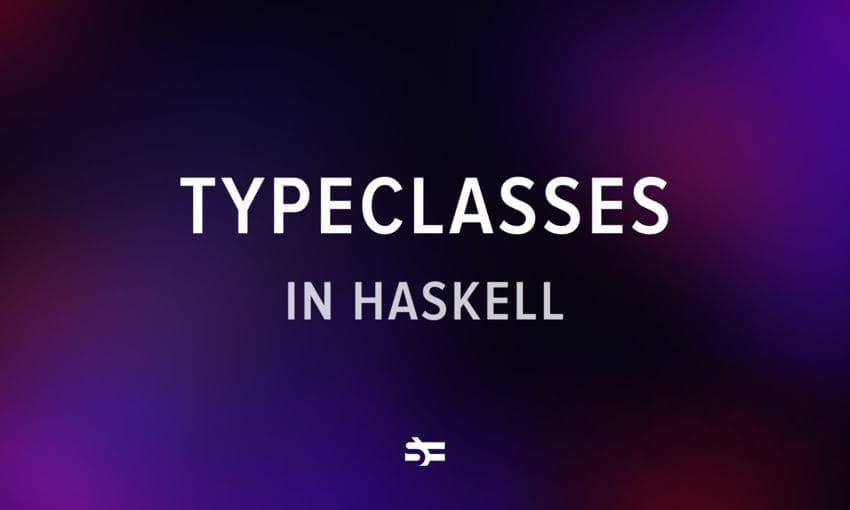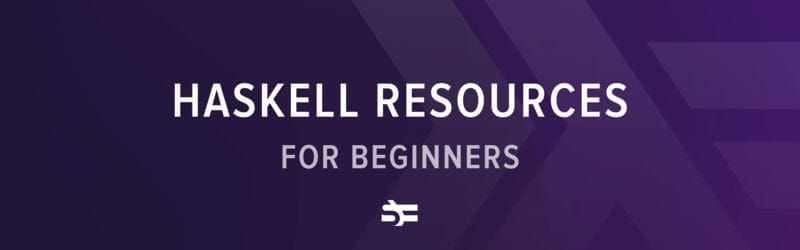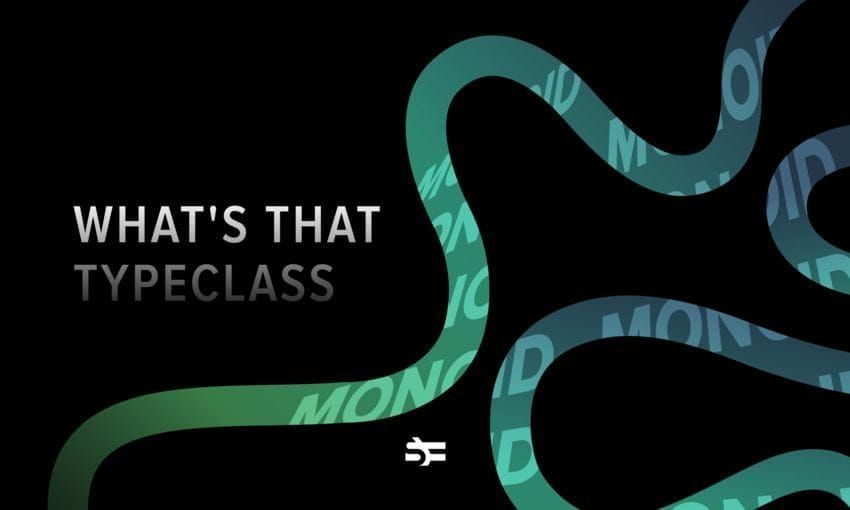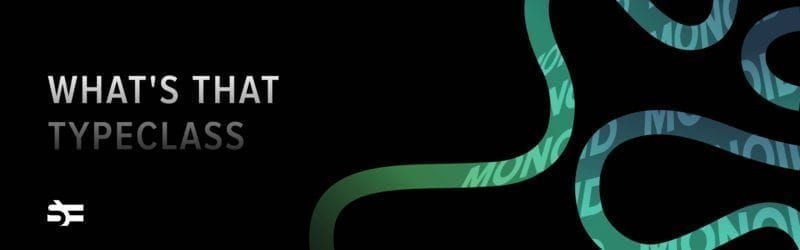# Algebraic Data Types in Haskell

Most programming languages have a way to make compound data types. In Haskell, we can do that via algebraic data types. Even though the name might sound scary at first, it’s simply a way to construct types.

This article will introduce you to the concept of algebraic data types and show you how to use them.

• what product and sum types are;
• why algebraic data types are called algebraic;
• how to use common Haskell ADTs such as Maybe and Either;
• why functions are called exponential types.

If you want to watch this article as a 10-minute video, you can do that on our YouTube channel.

## Product types

### Making custom data types in Haskell

Let’s start by creating a data type for a 2-dimensional point.

New data types are created via the data keyword. To create a Point data type, we need to provide a type constructor (the name of our type) and a data constructor (used to construct new instances of the type), followed by the types our type will contain.

--                
data Point = Point Double Double
deriving (Show, Eq)

-- : Type constructor.
-- : Data constructor.
-- : Types wrapped.


A few notes on this piece of code.

First of all, there’s a difference between the type constructor and the data constructor. In our example, they are called the same, but they could have been Point and Point2D, for example. This frequently confuses beginners.

 Type constructor Data constructor Name of the type. Used to construct an instance of a type. Each type can have only one type constructor. Each type can have multiple data constructors (in case of sum types).

Second, adding deriving (Show, Eq) to the type definition above makes it possible to print values of the type and to compare them for equality. You can read more about deriving in this blog post.

Let’s play with our Point type in GHCi.

We can create new values of this type via the data constructor.

*Main> a = Point 3 4
*Main> a
Point 3.0 4.0


And we can create functions that pattern match on constructors and values inside them.

*Main> distance (Point x1 y1) (Point x2 y2) = sqrt ((x1 - x2) ^ 2 + (y1 - y2) ^ 2)
*Main> a = Point 3 4
*Main> b = Point 1 2
*Main> distance a b
2.8284271247461903


### Definition of a product type

We call Point (and all types with a similar structure) a product type. All product types combine multiple elements that are in the data structure at the same time. It’s the same as saying that you need this type and that type.

### Polymorphic data types

Our previously created Point data type can contain only double-precision floats.

In some cases, we would want it to work with other numbers as well. If so, we need to make it polymorphic (able to work with multiple different data types).

data PPoint a = PPoint a a
deriving (Show, Eq)


Here, we provide the type constructor with a type variable a, which we can later use in the definition of our type. In contrast to our previous Point type, PPoint is a type that needs to be “completed” by providing it with a concrete type.

To better illustrate this fact, we can look at the kinds of both functions. While it is not possible to fully explain kinds in this article, you can think of them as type signatures for types.

As we can see, Point is a concrete type.

*Main> :kind Point
Point :: *


In contrast, PPoint is a function that takes a type and returns a concrete type.

*Main> :kind PPoint
PPoint :: * -> *


Another typical example of a polymorphic product type is the tuple type.

*Main> :info (,)
type (,) :: * -> * -> *
data (,) a b = (,) a b


It takes two types – a and b – and returns a type that has a in the first slot and b in the second slot.

### Records

The individual types of our Point type are not named. While it doesn’t really add any difficulty right now, working with something like Person String String String String can be confusing.

An alternative is to use records, which have field labels.

data Point = Point
{ x :: Double
, y :: Double
}
deriving (Show, Eq)

*Main> a = Point 3 4
*Main> a
Point {x = 3.0, y = 4.0}


Records also provide us with getter functions for free. The names of those getters are the same as the field names.

*Main> x a
3.0
*Main> y a
4.0


You can update a record by providing the fields you want to update (rest will stay the same).

*Main> b = a {x = 4}
*Main> b
Point {x = 4.0, y = 4.0}


And you can put these two things together to create functional record updates.

*Main> moveUp point = point {y = y point + 1}
*Main> c = moveUp a
*Main> c
Point {x = 3.0, y = 5.0}


Of course, you can also work with records via pattern matching as with basic product types.

*Main> getX (Point x _) = x
*Main> getX a
3.0


## Sum types

There’s another flavor of types – sum types – that lists several possible variants a type could have. You might have encountered something similar under the name of enums or union types.

The simplest example of a sum type is Bool.

--          
data Bool = False | True

-- : Type constructor.
-- [2, 4]: Data constructors.
-- : The pipe operator that separates data constructors.


Bool can be constructed by either True or False.

We can make functions such as a negation that work on the values of Bool.

neg :: Bool -> Bool
neg True = False
neg False = True


There are a lot of sum types in the wild that you wouldn’t even necessarily recognize as such. While it is not defined that way, an Int can be thought of as the enumeration of all the entries in [-2^29 .. 2^29-1], for example.

A more nontrivial example of a sum type would be a data type that fits both a 2-dimensional and a 3-dimensional point.

data Point = Point2D Double Double | Point3D Double Double Double
deriving (Show, Eq)


Now we can write a function that accepts both types of points by pattern matching on the data constructors.

pointToList :: Point -> [Double]
pointToList (Point2D x y) = [x, y]
pointToList (Point3D x y z) = [x, y, z]


Here’s an example of its usage:

*Main> a = Point2D 3 4
*Main> b = Point3D 3 4 5
*Main> pointToList a
[3.0,4.0]
*Main> pointToList b
[3.0,4.0,5.0]


### Definition of a sum type

Like product types, sum types are a way of putting together basic types to create a more complex one. But in comparison to product types, only one of those types can be present in any given instance of the type.

In other words, using a sum type is like saying that you need type a or type b: “I need True or False”, “I need a 2D point or a 3D point”, etc.

## Product types vs. sum types

Here’s a small table to help you remember the differences between these two groups of types.

 Product types Sum types Example data (,) a b = (,) a b data Bool = False | True Intuition Give me a and b Give me a or b

## Algebraic data types

So why are these types called product and sum types? Let’s get into it.

If you remember your school math lessons, you worked with numbers ($1$, $2$, $3$, etc.), variables ($x$, $y$, $z$, etc.), and operators ($+$, $-$, $*$, etc.). In algebraic data types, our numbers are the number of possible values a type can have and our operators are | and data constructors.

### Summing types

If we use | in the definition of a type, the type can have a value from the values of types on either side of the operator. As such, the amount of possible values it has is the sum of the amount of values those types have.

For example, False contains only one value. True also contains only one value. Bool = False | True contains $1 + 1$ values. If we add an Unknown value to Bool, we will have a type with three possible values, and so on.

### Multiplying types

If we use a data constructor, our type can have all the possible combinations of the sets of values we provide. As such, the amount of possible values it has is the product of the amount of values those types have.

For example, if our type consists of two booleans, such as whether a person checked in for both parts of a return flight, it will have $2 * 2 = 4$ possible values.

data CheckedInStatus = CheckedInStatus Bool Bool


Possible values of CheckedInStatus:

True True
True False
False True
False False


### Definition of an algebraic data type

By putting together sum and product types, we can construct elaborate types out of simple building blocks.

And this is what algebraic data types work with. They are a collection of one or more data constructors, composed with the | operator. In other words, they are a sum of products.

--                                          
data Point = Point2D Double Double | Point3D Double Double Double
-- The Point data type is a sum () of products (, ).


Now, let’s look at two commonly used ADTs in Haskell: Maybe and Either.

### Maybe

First ADT we’ll cover is Maybe, which you might have encountered in other languages as Optional.

*Main> :info Maybe
type Maybe :: * -> *
data Maybe a = Nothing | Just a


Sometimes, a function might not be able to return a value for a certain input. In that case, we can use the Maybe type. It has two possible data constructors: Just or Nothing. If the function succeeds, we wrap the result in Just. Otherwise, we return Nothing, which symbolizes something similar to null.

For example, Prelude has a scary function called head, which works on lists but not all of them.

In case we call it with an empty list, we’ll get an exception:

*Main> head []


We can make it give a result for each input by pattern matching on the contents of the list and returning Nothing in the case of an empty list.

safeHead :: [a] -> Maybe a
safeHead (x : _) = Just x

*Main> safeHead []
Nothing
Just 1


All in all, you can think of Maybe as a safer alternative to null.

### Either

Now, what if you want to know what made the function fail?

In that case, there is another data type that we can use – Either. It functions similarly to what is called Result in other languages.

*Main> :info Either
type Either :: * -> * -> *
data Either a b = Left a | Right b


In contrast to Maybe, it can store something on the left side, such as an error message.

Let’s rewrite our safeHead function with Either.

safeHead :: [a] -> Either String a
safeHead (x : _) = Right x

*Main> safeHead []
Right 1


All in all, you can think of Either as a safer alternative to exceptions.

## Exponential types (functions)

To blow your mind a little bit in the end: functions can also add to our “type algebra” since they also have types.

Imagine we have a data type for traffic lights.

data Light = Green | Yellow | Red


How many possible values are in the type Light -> Bool? (One can imagine that they encode all possible rules for when is it legal to cross the street.)

Let’s try to write them all out.

• True if it is Green, False if it is Yellow or Red.
• True if it is Green or Yellow, False if it is Red.
• True if it is Green, Yellow, or Red.
• True if it is Yellow or Red, False if it is Green.
• True if it is Red, False if it is Green or Yellow.
• False if it is Green, Yellow, or Red.
• True if it is Green or Red, False if it is Yellow.
• True if it is Yellow, False if it is Green or Red.

The final number is $8$, or $2^3$.

Turns out, if we have two types $a$ and $b$ with the amount of values inside those types being $|a|$ and $|b|$, respectively, then there are $|b|^ {|a|}$ functions in the set of possible functions from $a$ to $b$.

## Conclusion

In this article, we explored common ways of defining your own data types in Haskell. We looked at product types and sum types and how they work together to create algebraic data types. We also looked at common data types such as Maybe and Either and saw how functions are exponential data types.

Haskell’s type system is large and enables you to be very expressive, so there are a lot of things that we didn’t cover in our blog post, such as newtypes.

## Exercises

In case you want some practice with algebraic data types, here are a couple of quick exercises.

1. Create a data type called Person that stores a person’s full name, address, and phone number. Create a function for getting a person’s name and a function for changing their phone number.

2. Convert the data type created in exercise 1 to a record.

3. Given a data type for days of the week: data Day = Monday | Tuesday | Wednesday | Thursday | Friday | Saturday | Sunday, write two functions:

• isWednesday, which takes a day of the week and returns True if it’s Wednesday and False otherwise.
• nextDay, which takes a day of the week and returns the day of the week that comes after it.
4. Recall the Maybe data type we covered earlier. Write a ‘tail’ function for a list with the type signature of safeTail :: [a] -> Maybe [a]. It should take a list and return the list without the first element, wrapped in Just. In case that is not possible, it should return Nothing.

Some examples of its behavior:

* safeTail [ ] -> Nothing
* safeTail  -> Just []
* safeTail [1,2,3,4,5] -> Just [2,3,4,5]


## Appendix

Here’s a handy table of some of the types we have covered in this article and how to compute the cardinality (how many members the type has) of those types, assuming that the cardinalities of their components are known.

Note: $|a|$ notes the cardinality of the type $a$ in the table.

 Name Haskell Cardinality Bool data Bool = False | True $1 + 1$ Maybe data Maybe a = Nothing | Just a $1 + |a|$ Either data Either a b = Left a | Right b $|a| + |b|$ Tuple (a, b) $|a| * |b|$ Function a -> b $|b| ^{|a|}$ 2D point data Point = Point Double Double $|Double| * |Double|$ 2D or 3D point data Point = Point2D Double Double | Point3D Double Double Double $|Double| * |Double| + |Double| * |Double| * |Double|$